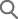• 雀盔丛狼 酒捞叼客 菩胶况靛甫 涝仿秦 林技夸.
• 雀盔捞 酒聪矫搁 酒贰 [雀盔啊涝]阑 喘矾 雀盔啊涝阑 秦林矫扁 官而聪促.

HONGGIDONG

honggildong@naver.com

• * 档肺疙, 扒拱疙, 瘤锅俊 措秦 烹钦八祸捞 啊瓷钦聪促.
(抗: 馆器措肺 58, 惫赋吝居冠拱包, 伙己悼 25)
• * 狐弗 八祸阑 困秦 林家, 档肺疙, 扒拱疙, 扁鸥矫汲拱疙(瘤窍枚开荤疙/扁鸥矫汲拱 殿)阑 沥犬窍霸 涝仿窍
咯 林技夸.
• * 距侥栏肺 八祸窍矫绰 版快 八祸捞 力措肺 登瘤 臼阑 荐 乐嚼聪促.
(抗: 馆器, 惫吝, 伙己)• 酒捞叼/厚剐锅龋甫 镭栏继唱夸? 酒贰 亲格阑 沥犬洒 涝仿秦 林矫扁 官而聪促.

E-mail

### Measuring Systemic Risk in the Banking Sector Using the Conditional Probability of Default

• Seung Hwan Lee
The 2007~2009 global financial crisis has highlighted the danger of systemic risk, largely caused by interconnectedness and externality among financial institutions. Generally, systemic risk is a difficult concept to define and measure accuately, making it even more challenging for policy makers to effectively address the issue. Although no clear consensus has been established on a formal definition of systemic risk, it usually denotes the risk related to all the potential results followed by the collapse of a whole financial system, consisting of many interacting financial institutions. In order to effectively prevent such systemic risk, it is necessary to first develop a reliable method to measure such risk. The previous studies on the systemic risk can be divided into two structural approaches: using contingent claims analysis (Lehar, 2005, Gray, Merton, and Bodie, 2008, and Gray and Jobst, 2009) and reduced- form approaches focusing on the statistical tail behavior of financial institutions’ asset returns (Adrian and Brunnermeier, 2009, and Chan-Lau, 2010). In this paper, we propose a new method to gauge systemic risk: the conditional probability of default (CoPD), which is derived from the joint probability distributions of banks’ asset returns. In order to obtain CoPD, we first estimate the joint probability distributions of asset returns and the default points of banks in the system. Since the market values of bank assets are unobservable, we estimate asset returns using stock prices and debt maturity structures following Merton (1974) and Crosbie and Bohn (2003). To maximize the consistency between asset values and asset volatilities, we estimate them using the recursive exponentially weighted moving average algorithm developed by Lee (2010). In doing so, we assume that a bank is in default if the market value of its assets is less than the default point, which is estimated based on the debt maturity structure of banks. We define CoPD as a default probability of a bank, conditional on other banks being in default. The difference between CoPD and unconditional PD, CoPD, contains useful information on systemic risk.CoPD?深? denotes the change in default probability of bank i when bank j goes bankrupt. The stronger the interdependence between bank i and bank j gets, the higher CoPD?深? becomes. This method allows us to develop systemic risk indicators which measure how much the default possibility of banks increases when other banks in the system go bankrupt. The systemic risk indicator (SRI) can be calculated based on the n】n CoPD matrix, whose element CoPD?深? denotes the difference between CoPD?深? and unconditional PD ? . The overall systemic risk may be captured by the mean value of all off-diagonal elements in the matrix. SRI measures the increase in default probability of a bank on average when a bank in the system falls default. If there are no direct and indirect connections between banks, SRI approaches 0. The stronger the interdependence within the banking system gets, the higher SRI will be. In order to measure how much the default probabilities of other banks increase when the bank goes bankrupt, we also develop the systemic importance indicator (SII) of each individual bank as well as the systemic vulnerability indicator (SVI), which are supplementary information of the systemic risk indicator. We may calculate SII as the column mean of the CoPD matrix. On the other hand, the systemic vulnerability indicator of an individual bank measures how much the default probability of the bank increases when other banks go bankrupt. Thus, SVI can be calculated as the row mean of the CoPD matrix. Meanwhile, Adrian and Brunnermeier (2009) have proposed a popular systemic risk measure: CoVaR, which may evaluate the systemic risk contribution of an individual bank. However, this method entails some serious measurement errors on tail dependence. The concept of tail dependence generates a measure for extreme co-movements in the lower and upper tail of asset return distributions, respectively. A reliable systemic risk measure must correctly capture the lower tail dependence among the involved banks. Simulation results suggest that CoVaR underestimates the systemic risk if lower tail dependence is significant, whereas it overestimates the systemic risk if upper tail dependence is strong. The Monte-Carlo experiments show that CoPD can accurately address the problem with CoVaR on tail dependence. The CoPD may also reflect the information about the financial strength of banks. In this study, we calculate the systemic risk indicators for six Korean domestic banks by applying our method. The empirical results show that the systemic risk indicator of the Korean banking system had remained stable for a long period of time after the 2003 credit card crisis until the sub-prime mortgage crisis broke out (2007~2009). Since then the systemic risk of the Korean banking system has dramatically increased again. Secondly, large banks’ systemic importance is considerably high, while low-capital banks are systemically vulnerable. Finally, the BIS capital ratio and the FX liquidity ratio have changed in the opposite direction of the systemic risk indicator with a lead of two or three quarters.